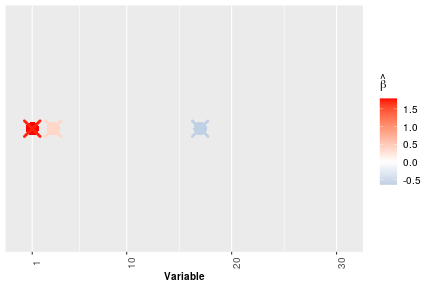Introduction

The package \textsf{GlarmaVarSel} provides functions for performing variable selection approach in sparse GLARMA models, which are pervasive for modeling discrete-valued time series. The method consists in iteratively combining the estimation of the autoregressive moving average (ARMA) coefficients of GLARMA models with regularized methods designed to perform variable selection in regression coefficients of Generalized Linear Models (GLM). For further details on the methodology we refer the reader to .

We describe the GLARMA model for a single time series with additional covariates. Given the past history $$\mathcal{F}_{t-1} = \sigma(Y_s, s\leq t-1)$$, we assume that \begin{equation}Yt | \mathcal{F}{t-1} \sim \mathcal{P}(\mut{\star}), \label{eq1} \end{equation} where $$\mathcal{P}(\mu)$$ denotes the Poisson distribution with mean $$\mu$$. In (\ref{eq1}) \begin{equation} \mu_t{\star} = \exp (W_t{\star}) \quad \text{with} \quad W_t{\star} = \beta_0{\star} + \sum{i=1}p \betai{\star} x{t,i} + Zt{\star}, \label{eq2} \end{equation} where the $x{t,i}$'s are the $$p$$ regressor variables at time $$t$$ with $$p \geq 1$$, $$\pmb{\beta}^{\star} = (\beta_{0}^{\star}, \dots, \beta_p^{\star})$$ is the vector of regressor coefficients, $$\pmb{\gamma}^{\star} = (\gamma_{0}^{\star}, \dots, \gamma_{q}^{\star})$$ is an absolutely summable series and\begin{equation} Zt{\star} = \sum{j=1}q \gamma{\star}_j E{\star}_{t-j} \quad \text{with} \quad E_t{\star} = \frac{Y_t - \mu_t{\star}}{\mu_t{\star}} = Y_t \exp(-W_t*) -1. \label{eq3} \end{equation} Here $$E_t^{\star} = 0$$ for all $$t \leq 0$$ and $$1 \leq q \leq \infty$$. When $$q=\infty$$, $$Z^{\star}_t$$ satisfies the ARMA-like recursion in (\ref{eq3}), because causal ARMA can be written as MA process of infinite order. The vector $$\pmb{\beta}^{\star}$$ is assumed to be sparse, \textit{i.e.} a majority of its components is equal to zero. The goal of the \textsf{GlarmaVarSel} package is to retrieve the indices of the nonzero components of $$\pmb{\beta}^{\star}$$, also called active variables, from the observations $$Y_1, \dots, Y_n$$.

Data

We load the dataset of observations \verb|Y| with size $$n=50$$ provided within the package.

data(Y)

The number of regressor variables $$p$$ is equal to 30. Data \verb|Y| is generated with $$\pmb{\gamma}^{\star} = (0.5)$$ and $$\pmb{\beta^{\star}}$$, such that all the $$\beta_i^{\star}=0$$ except for three of them: $$\beta_1^{\star}=1.73$$, $$\beta_3^{\star}=0.38$$ and $$\beta_{17}^{\star}=-0.64$$. The design matrix $$X$$ is built by taking the covariates in a Fourier basis.

Initialization

We initialize $$\pmb{\gamma^{0}} = (0)$$ and $$\pmb{\beta^{0}}$$ to be the coefficients estimated by \textsf{glm} function:

gamma0 = c(0)

glm_pois<-glm(Y~t(X)[,2:(p+1)],family = poisson)
beta0<-as.numeric(glm_pois$coefficients) Estimation of $$\pmb{\gamma^{\star}}$$ We can estimate $$\pmb{\gamma^{\star}}$$ with the Newton-Raphson method. The output is the vector of estimation of $$\pmb{\gamma^{\star}}$$. The default number of iterations \verb|n_iter| of the Newton-Raphson algorithm is 100. gamma_est_nr = NR_gamma(Y, X, beta0, gamma0, n_iter=100) gamma_est_nr ##  0.4121483 This estimation is obtained by taking initial values $$\pmb{\gamma^{0}}$$ and $$\pmb{\beta^{0}}$$, which will improve once we substitute the initial values by $$\pmb{\hat{\gamma}}$$ and $$\pmb{\hat{\beta}}$$ obtained by \verb|variable_selection| function. Variable selection We perform variable selection and obtain the coefficients which are estimated to be active and the estimates of $$\pmb{\gamma^{\star}}$$ and $$\pmb{\beta^{\star}}$$. We take the number of iterations of the algorithm \verb|k_max| equal to 2. We take \verb|min| method, which corresponds to the stability selection method with minimal $$\lambda$$, where \verb|threshold| is equal to 0.7 and the number of replications \verb|nb_rep_ss| $$=1000$$. For more details about stability selection and the choice of parameters we refer the reader to . The function supports parallel computation. To make it work, users should download the package \textsf{doMC}, which is not supported on Windows platforms. result = variable_selection(Y, X, gamma0, k_max = 2, n_iter = 100, method = "min", nb_rep_ss = 1000, threshold = 0.7, parallel = FALSE, nb.cores = 1) beta_est = result$beta_est
Estim_active = result$estim_active gamma_est = result$gamma_est
## Estimated active coefficients:  1 3 17
## Estimated gamma:  0.5083098

Illustration of the estimation of $$\pmb{\beta^{\star}}$$

We display a plot that illustrates which elements of $$\pmb{\beta^{\star}}$$ are selected to be active and how close the estimated value $$\hat{\beta_i}$$ is to the actual values $$\beta^{\star}_i$$. True values of $$\pmb{\beta^{\star}}$$ are plotted in crosses and estimated values are plotted in dots.

# First, we make a dataset of estimated betas
beta_data = data.frame(beta_est)
colnames(beta_data) <- "beta"
beta_data$Variable = seq(1, (p + 1), 1) beta_data$y = 0
beta_data = beta_data[beta_data$beta != 0, ] # Next, we make a dataset of true betas beta_t_data = data.frame(beta) colnames(beta_t_data) <- "beta" beta_t_data$Variable = seq(1, (p + 1), 1)
beta_t_data$y = 0 beta_t_data = beta_t_data[beta_t_data$beta != 0, ]
# Finally, we plot the result
plot = ggplot() + geom_point(data = beta_data, aes(x = Variable,
y = y, color = beta), pch = 16, size = 5, stroke = 2) +
geom_point(data = beta_t_data, aes(x = Variable, y = y,
color = beta), pch = 4, size = 6, stroke = 2) +
midpoint = 0, low = "steelblue", mid = "white",
high = "red") + scale_x_continuous(breaks = c(1,
seq(10, (p + 1), 10)), limits = c(0, (p + 1))) + scale_y_continuous(breaks = c(),
limits = c(-1, 1)) + theme(legend.title = element_text(color = "black",
size = 12, face = "bold"), legend.text = element_text(color = "black",
size = 10)) + theme(axis.text.x = element_text(angle = 90),
axis.text = element_text(size = 10), axis.title = element_text(size = 10,
face = "bold"), axis.title.y = element_blank())
plotAs we can see from the plot, all the zero coefficients are estimated to be zero and all non-zero coefficients are estimated to be non-zero. Moreover, the estimates also correctly preserved the sign of the coefficients.

References

 M. Gomtsyan, C. Lévy-Leduc, S. Ouadah and L. Sansonnet. “Variable selection in sparse GLARMA models”, arXiv:arXiv:2007.08623v1